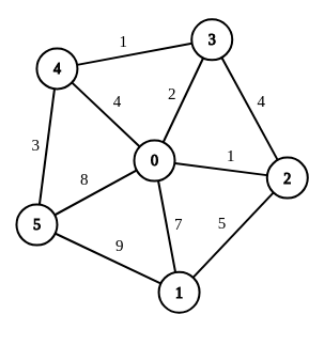시간 제한 메모리 제한 제출 정답 맞은 사람 정답 비율
1 초 256 MB 4 2 2 50.000%

문제

There is a graph with N + 1 vertices numbered 0 to N. For 1 ≤ i ≤ N, an edge with capacity Ai connects vertices 0 and N. For 1 ≤ i ≤ N, an edge with capacity Bi connects vertices i and ((i mod N) + 1). There are no other edges in the graph. All edges are undirected.

A flow in a graph from a source vertex s to a sink vertex t assigns to each edge e = (v1, v2) a direction (either v1 → v2 or v2 → v1) and a number Fe such that 0 ≤ Fe ≤ Ke, where Ke is the capacity of edge e. We say that there are Fe units of flow in the specified direction, out of v1 or v2, and into the other vertex. Every flow must satisfy the constraint that for each vertex v except the source and sink, the sum of the flows out of v equals the sum of the flows into v. The maximum flow from s to t is the maximum over all flows from s to t of the sum of flows out of s minus the sum of flows into s.

Let Ci be the maximum flow from vertex 0 to vertex i. Find the value of C1 + · · · + CN.

Sequences A and B will not be given directly. Instead N, A1, B1 and integers P, Q, W, X are given. Then for i > 1:

• Ai = (PAi−1) mod Q
• Bi = (WBi−1) mod X

입력

Line 1 contains one integer N (2 ≤ N ≤ 220).

Line 2 contains three integers A1, P, Q (1 ≤ A1, P, Q ≤ 229).

Line 3 contains three integers B1, W, X (1 ≤ B1, W, X ≤ 229).

출력

Print one line with one integer, the value of C1 + · · · + CN.

예제 입력 1

5
7 2 13
5 3 11


예제 출력 1

64


힌트

For the first example, A = [7, 1, 2, 4, 8], B = [5, 4, 1, 3, 9], C = [20, 9, 7, 8, 20]. The graph is shown below.